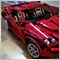# Script to calculate the risk of all open orders. Problems with JPY pairs.383

Hi guys,

can you tell me what's wrong with my calculations? Works fine on 5-digit-pairs as well as on metals but to get the correct values for JPY pairs I need to divide them by 100.

But why? I thought my calculation is correct for every pair.

```void OnStart()
{
double openRisk=0;
for (int i=OrdersTotal(); i>=0; i--) {
if (OrderSelect(i, SELECT_BY_POS)) {
if (OrderCloseTime()==0) {
if (OrderType()==OP_SELL) {
Print(OrderSymbol()+": "+(OrderOpenPrice()-OrderStopLoss())*OrderLots()*MarketInfo(OrderSymbol(), MODE_LOTSIZE)*MarketInfo(OrderSymbol(), MODE_TICKVALUE)+OrderCommission());
openRisk=openRisk+(OrderOpenPrice()-OrderStopLoss())*OrderLots()*MarketInfo(OrderSymbol(), MODE_LOTSIZE)*MarketInfo(OrderSymbol(), MODE_TICKVALUE)+OrderCommission();
}
else if (OrderType()==OP_BUY) {
Print(OrderSymbol()+": "+(OrderStopLoss()-OrderOpenPrice())*OrderLots()*MarketInfo(OrderSymbol(), MODE_LOTSIZE)*MarketInfo(OrderSymbol(), MODE_TICKVALUE)+OrderCommission());
openRisk=openRisk+(OrderStopLoss()-OrderOpenPrice())*OrderLots()*MarketInfo(OrderSymbol(), MODE_LOTSIZE)*MarketInfo(OrderSymbol(), MODE_TICKVALUE)+OrderCommission();
}
}
}
}
Alert(openRisk);
}```403

Marbo:

Hi guys,

can you tell me what's wrong with my calculations? Works fine on 5-digit-pairs as well as on metals but to get the correct values for JPY pairs I need to divide them by 100.

But why? I thought my calculation is correct for every pair.

I've always been using this formula to compute risk:

`PriceDifference/TickSize*LotSize*TickValue`383

Seng Joo Thio:

I've always been using this formula to compute risk:

I have modified my script according to your formula but unfortunately it doesn't work:

```void OnStart()
{
double openRisk=0;
for (int i=OrdersTotal(); i>=0; i--) {
if (OrderSelect(i, SELECT_BY_POS)) {
if (OrderCloseTime()==0) {
if (OrderType()==OP_SELL) {
Print(OrderSymbol()+": "+(OrderOpenPrice()-OrderStopLoss())/OrderLots()*MarketInfo(OrderSymbol(), MODE_TICKVALUE)*MarketInfo(OrderSymbol(), MODE_TICKSIZE));
openRisk=openRisk+(OrderOpenPrice()-OrderStopLoss())/OrderLots()*MarketInfo(OrderSymbol(), MODE_TICKVALUE)*MarketInfo(OrderSymbol(), MODE_TICKSIZE);
}
else if (OrderType()==OP_BUY) {
Print(OrderSymbol()+": "+(OrderStopLoss()-OrderOpenPrice())/OrderLots()*MarketInfo(OrderSymbol(), MODE_TICKVALUE)*MarketInfo(OrderSymbol(), MODE_TICKSIZE));
openRisk=openRisk+(OrderStopLoss()-OrderOpenPrice())/OrderLots()*MarketInfo(OrderSymbol(), MODE_TICKVALUE)*MarketInfo(OrderSymbol(), MODE_TICKSIZE);
}
}
}
}
Alert(openRisk);
}```403

Marbo:

I have modified my script according to your formula but unfortunately it doesn't work:

Your sequence is wrong.

`(PriceDifference/TickSize)*LotSize*TickValue`

Divide tick size first, then multiply result with LotSize and TickValue.383

Seng Joo Thio:

Your sequence is wrong.

Divide tick size first, then multiply result with LotSize and TickValue.

Obviously you are a maths genius! :)

It works perfect. Thank you very much!

To add comments, please log in or register